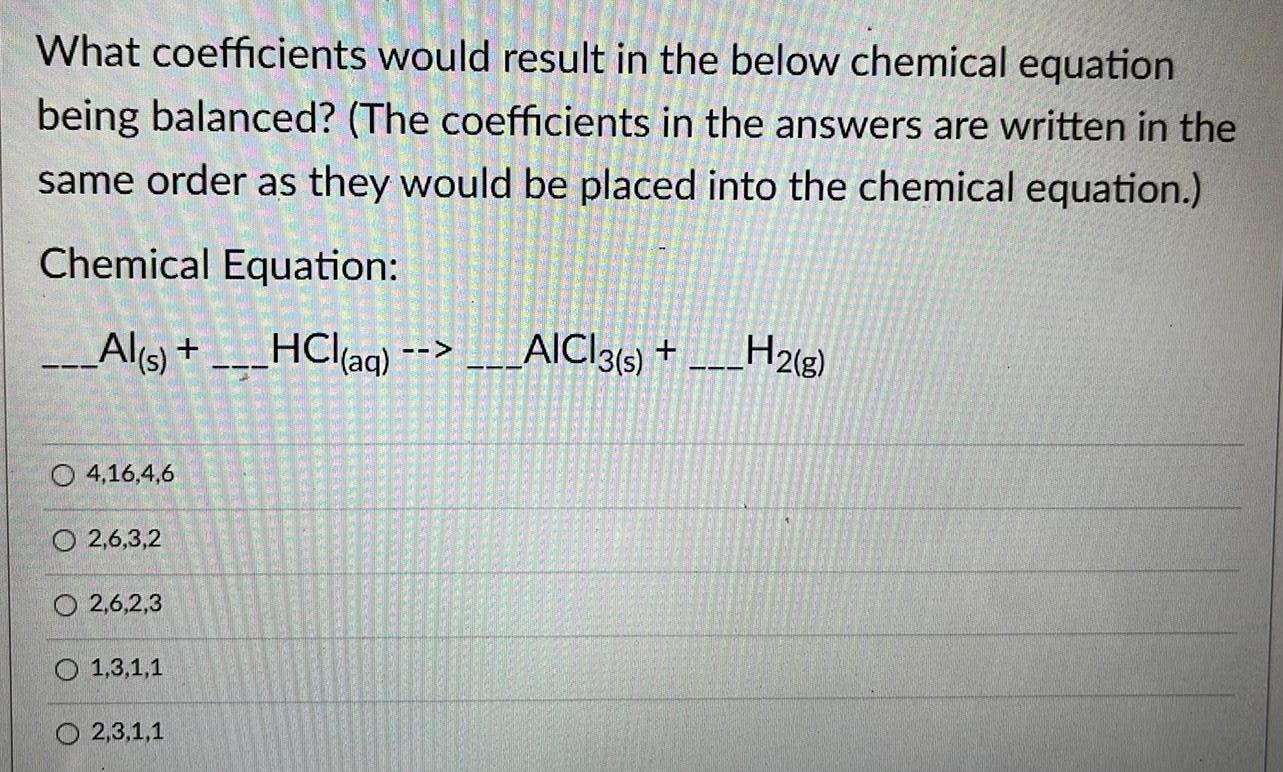Question:

# What coefficients would result in the below chemical

Last updated: 8/7/2022What coefficients would result in the below chemical equation being balanced? (The coefficients in the answers are written in the same order as they would be placed into the chemical equation.) Chemical Equation: _Al(s) + HCl(aq) --> ___AICI3(s) +___H2(g) 4,16,4,6 2,6,3,2 2,6,2,3 1,3,1,1 O2,3,1,1